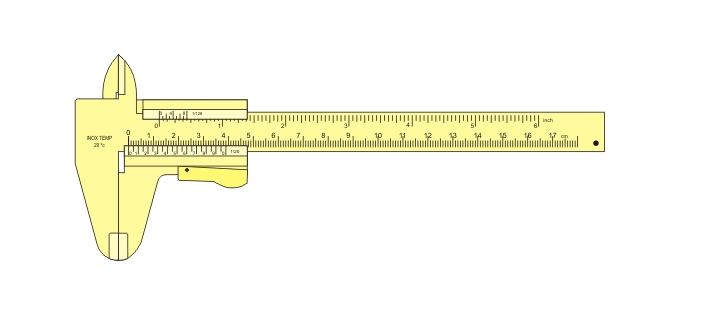# Class XI Physics Practical Exam Viva Voce

Hi Students!

The practical examinations are accompanied by viva voce by the external/internal examiner to ensure the genuineness of their work and to analyse the understanding of the subject by the students.

So it is important to prove yourself by performing well in the viva voce.

The following questions will be of some help to prepare well for it. Please remember that the questions following the first is based on your answer to the first question. (However, there are some examiners who come with some questions written on their diary or paper and blindly ask from them. For them, please prepare the questions which are printed on the practical manual.)

Questions based on screw gauge experiments

1. Define leastcount of a screw gauge
2. Define pitch
3. What is backlash error? How is it avoided?
4. What is zero error?  How is it determined?
5. When do you say that the zero error is positive or negative?
6. What are random errors? How can you minimise them?
7. What is the use of ratchet in a screw gauge?

Questions based on vernier calipers experimentsusing the vernier caliper to measure a nut (Photo credit: Wikipedia)
1. Define vernier constant?
2. What is the principle of a vernier?
3. How is the least count of a vernier calipers determined?
4. What are the common sources of error occurring in using a vernier?
5. What are the precautions to be observed while using vernier?
6. What is parallax error? How can it be avoided?
7. What are the uses of a vernier calipers?
8. How will you determine the volume of an irregular glass plate using vernier?

Questions based on simple pendulum experiments

1. What is a simple pendulum?
2. Define time period of a simple pendulum.
3. Define effective length of a simple pendulum.
4. What are the factors affecting the time period of a simple pendulum?
5. How does altitude from the surface of earth affect the time period of a simple pendulum?
6. What is a seconds pendulum?
7. What is the effective length of a seconds pendulum?
8. Define SHM.
9. Why should the oscillations of the simple pendulum must be of small amplitude?
10. What are the precautions to be observed while doing the simple pendulum experiment?
11. What is the shape of LT^2 graph?
12. What is the shape of L-T graph?
13. Define point of oscillation of a simple pendulum.

Questions based on Hooke’s Law/Spring constant/helical spring experiments

1. State Hooke’s Law
2. Define spring constant
3. What is restoring force?
4. What is the shape of load extension graph?
5. How do you find the spring constant from the load – extension graph?
6. Define elasticity?
7. What do you mean by modulus of elasticity?
8. Which is more elastic – steel of rubber? Why?

Questions based on parallelogram law/concurrent forces experiments

1. What are concurrent forces?
2. State parallelogram law of forces.
3. What do you mean by resultant of a no. of forces?
4. What do you mean by equilibrant?
5. What are the conditions for translational and rotational equilibrium?
6. State triangle law of forces.
7. Define force.
8. What are co-planar forces?
9. What is the minimum no. of non co-planar forces required to keep a body in equilibrium?
10. What is the minimum no. of non collinear forces required to keep a body in equilibrium?

Questions based on spherometer experiments